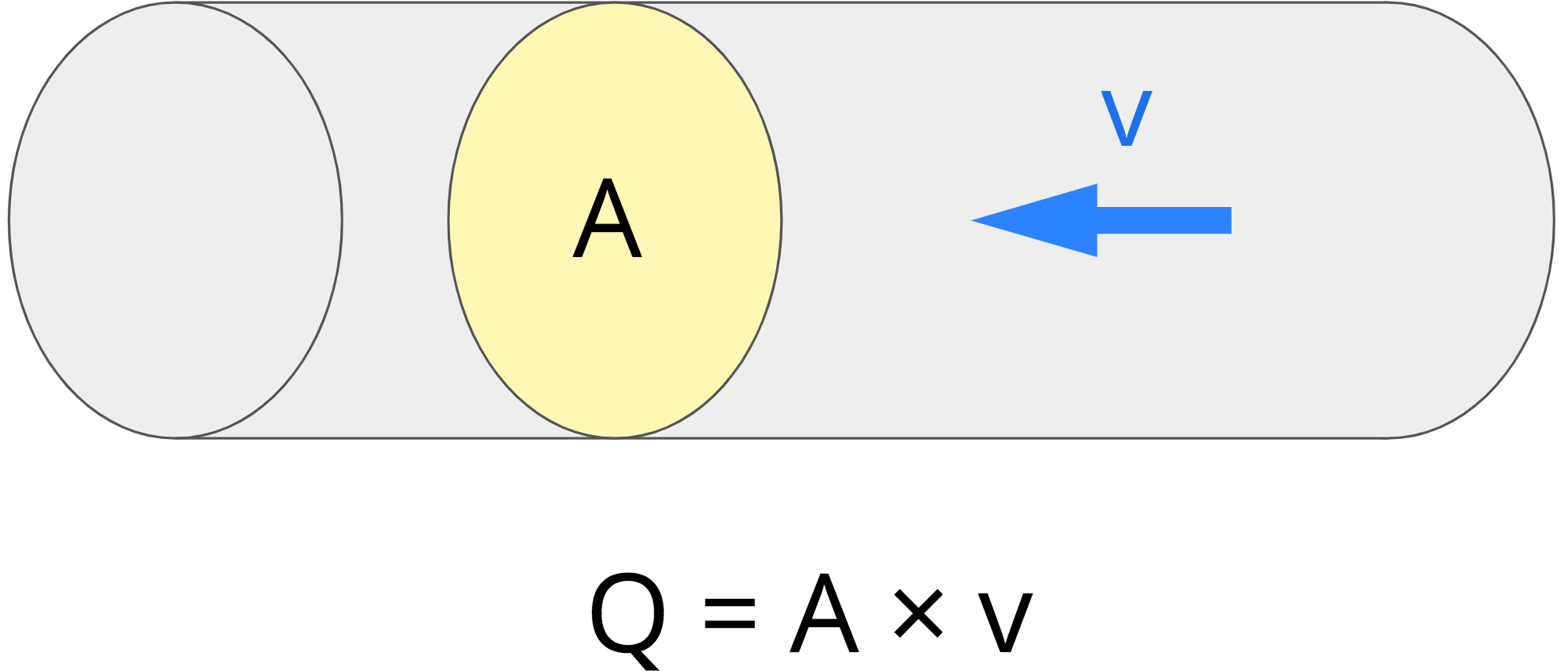# Flow Rate Calculator

Enter either the pipe diameter and velocity or the volume of fluid passing in a given time to calculate the volumetric flow rate.

ft³/sec

ft³/min

ft³/hr

gal/sec

gal/min

gal/hr

m³/sec

m³/min

m³/hr

l/sec

l/min

l/hr

## How to Calculate Flow Rate

Volumetric flow rate, also referred to as volume flow rate, flow rate, or volume velocity, is defined as the volume of fluid passing a point in a given unit of time. The SI unit of flow rate is cubic meters per second (m³/s).

Flow rate can be found by simply measuring the amount of fluid that fills a bucket in a given amount of time or using a formula.

### Flow Rate Formula

The flow rate of a fluid can be calculated using the volumetric flow rate equation:

Q = A × v

Thus, the volume flow rate Q is equal to the cross-sectional area of the pipe A times the velocity of the fluid v.To use the flow rate formula, substitute the cross-sectional area of the pipe and the velocity of the liquid. For a round pipe, the cross-sectional area can be found using the formula for circle area A = πr².

For example, let’s find the flow rate for the fluid passing through a 1″ diameter pipe at a velocity of 3 feet per second.

Start by finding the cross-sectional area of the pipe.

area = π × r²
area = 3.14 × (1 ÷ 2)²
area = 3.14 × 0.25
area = 0.785398 in²

Next, it will be easier to solve later if the velocity and the area are in the same units. Convert the area in square inches to square feet by dividing by 144.

A = 0.785398 in² ÷ 144
A = 0.00545 ft²

Finally, substitute the area and velocity in the flow rate equation and solve.

Q = 0.00545 in² × 3 ft/sec
Q = 0.01635 cubic ft/sec

Thus, the flow rate is 0.01635 cubic feet per second.

### Alternate Flow Rate Formula

An alternative formula to solve flow rate is:

Q = Vt

The volume flow rate Q is equal to the volume of liquid passed V divided by the time passed t.

To use this formula, you need to be able to measure the volume of liquid that passes in a given time.

For example, let’s find the flow rate for a garden hose that can fill a 5-gallon bucket in 3 minutes.

Q = 53
Q = 1.67 gal/min

Thus, the flow rate is 1.67 gallons per minute.

Try our unit rate calculator to find the rate of other units.

## References

1. Engineers Edge, Fluid Volumetric Flow Rate Equation, https://www.engineersedge.com/fluid_flow/volumeetric_flow_rate.htm
2. Chris Deziel, How to Calculate Flow Rates, Sciencing, https://sciencing.com/calculate-flow-rates-6199676.html